﻿ Reading & Wriring INI file from SD Micro - Netduino Plus 2 (and Netduino Plus 1) - Netduino Forums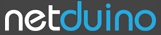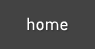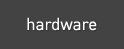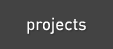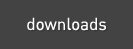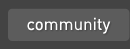The Netduino forums have been replaced by new forums at community.wildernesslabs.co. This site has been preserved for archival purposes only and the ability to make new accounts or posts has been turned off.# Reading & Wriring INI file from SD Micro

2 replies to this topic

### #1OneManDo

OneManDo

Posted 05 March 2012 - 04:05 PM

Here is a class for reading & writing INI file from/to Micro SD
It is very simple one... but does the work just fine ;-)
Any feedback will be nice to have.

Here is the source code:

```using System;
using System.IO;
using System.Collections;

namespace NetduinoPlusMonitor
{
public class CIni
{
public CIni()
{
_Sections = new Hashtable();
}

/// <summary>
/// Loads the Reads the data in the ini file into the IniFile object
/// </summary>
/// <param name="filename"></param>
public void Load(string FileName, bool append)
{
if (!append)
DeleteSections();

string Section = "";
string[] keyvaluepair;

using (FileStream inFileStream = new FileStream(Path.Combine("\\SD",FileName), FileMode.Open))
{
{

foreach(string line in lines)
{
if (line == string.Empty)
continue;
if (';' == line)
continue;

if ('[' == line && ']' == line[line.Length - 1])
Section = line.Substring(1, line.Length - 2);

else if (-1 != line.IndexOf("="))
{
keyvaluepair = line.Split(new char[] { '=' });
SetValue(Section, keyvaluepair, keyvaluepair);
}
}
inFileStream.Close();
}
}

}

/// <summary>
/// Used to save the data back to the file or your choice
/// </summary>
/// <param name="FileName"></param>
public void Save(string FileName)
{
using (FileStream outFileStream = new FileStream(Path.Combine("\\SD",FileName), FileMode.Create))
{
using (StreamWriter outStreamWriter = new StreamWriter(outFileStream))
{
foreach (object Sections in _Sections.Keys)
{
outStreamWriter.WriteLine("[" + Sections.ToString() + "]");
Hashtable keyvalpair = (Hashtable)_Sections[Sections];

foreach (object key in keyvalpair.Keys)
outStreamWriter.WriteLine(key.ToString() + "=" + keyvalpair[key].ToString());
}

outStreamWriter.Close();
outFileStream.Close();
}
}

}

public string GetValue(string Section, string key, string defkey = "")
{
key = key.ToLower();
Section = Section.ToLower();

Hashtable keyvalpair = (Hashtable)_Sections[Section];

if ((null != keyvalpair) && (keyvalpair.Contains(key)))
defkey = keyvalpair[key].ToString();

return defkey;
}
public float GetValue(string Section, string key, float defkey)
{
try { defkey = (float)double.Parse(GetValue(Section, key, defkey.ToString())); }
catch (Exception) { }
return defkey;
}
public double GetValue(string Section, string key, double defkey)
{
try { defkey = double.Parse(GetValue(Section, key, defkey.ToString())); }
catch (Exception) { }
return defkey;
}
public UInt64 GetValue(string Section, string key, UInt64 defkey)
{
try { defkey = UInt64.Parse(GetValue(Section, key, defkey.ToString())); }
catch (Exception) { }
return defkey;
}
public UInt32 GetValue(string Section, string key, UInt32 defkey)
{
try { defkey = UInt32.Parse(GetValue(Section, key, defkey.ToString())); }
catch (Exception) { }
return defkey;
}
public UInt16 GetValue(string Section, string key, UInt16 defkey)
{
try { defkey = UInt16.Parse(GetValue(Section, key, defkey.ToString())); }
catch (Exception) { }
return defkey;
}
public byte GetValue(string Section, string key, byte defkey)
{
try { defkey = byte.Parse(GetValue(Section, key, defkey.ToString())); }
catch (Exception) { }
return defkey;
}
public Int64 GetValue(string Section, string key, Int64 defkey)
{
try { defkey = Int64.Parse(GetValue(Section, key, defkey.ToString())); }
catch (Exception) { }
return defkey;
}
public Int32 GetValue(string Section, string key, Int32 defkey)
{
try { defkey = Int32.Parse(GetValue(Section, key, defkey.ToString())); }
catch (Exception) { }
return defkey;
}
public Int16 GetValue(string Section, string key, Int16 defkey)
{
try { defkey = Int16.Parse(GetValue(Section, key, defkey.ToString())); }
catch (Exception) { }
return defkey;
}

public void SetValue(string Section, string key, string value)
{
key = key.ToLower();
Section = Section.ToLower();

if (!_Sections.Contains(Section))

Hashtable keyvalpair = (Hashtable)_Sections[Section];

if (keyvalpair.Contains(key))
keyvalpair[key] = value;
else
}
public void SetValue(string Section, string key, float value)
{
SetValue(Section, key, value.ToString());
}
public void SetValue(string Section, string key, double value)
{
SetValue(Section, key, value.ToString());
}
public void SetValue(string Section, string key, byte value)
{
SetValue(Section, key, value.ToString());
}
public void SetValue(string Section, string key, Int16 value)
{
SetValue(Section, key, value.ToString());
}
public void SetValue(string Section, string key, Int32 value)
{
SetValue(Section, key, value.ToString());
}
public void SetValue(string Section, string key, Int64 value)
{
SetValue(Section, key, value.ToString());
}
public void SetValue(string Section, string key, char value)
{
SetValue(Section, key, value.ToString());
}
public void SetValue(string Section, string key, UInt16 value)
{
SetValue(Section, key, value.ToString());
}
public void SetValue(string Section, string key, UInt32 value)
{
SetValue(Section, key, value.ToString());
}
public void SetValue(string Section, string key, UInt64 value)
{
SetValue(Section, key, value.ToString());
}

public void DeleteSection(string Section)
{
Section = Section.ToLower();

if (_Sections.Contains(Section))
_Sections.Remove(Section);
}
public void DeleteSections()
{
_Sections.Clear();
}

private Hashtable _Sections = null;

}
}```

Edited by Stefan, 05 March 2012 - 04:19 PM.

### #2willgeorge

willgeorge

Posted 06 March 2012 - 08:00 AM

Here is a class for reading & writing INI file from/to Micro SD
It is very simple one... but does the work just fine ;-)
Any feedback will be nice to have.

Here is the source code:

```using System;
using System.IO;
using System.Collections;

namespace NetduinoPlusMonitor
{
public class CIni
{
public CIni()
{
_Sections = new Hashtable();
}

/// <summary>
/// Loads the Reads the data in the ini file into the IniFile object
/// </summary>
/// <param name="filename"></param>
public void Load(string FileName, bool append)
{
if (!append)
DeleteSections();

string Section = "";
string[] keyvaluepair;

using (FileStream inFileStream = new FileStream(Path.Combine("\\SD",FileName), FileMode.Open))
{
{

foreach(string line in lines)
{
if (line == string.Empty)
continue;
if (';' == line)
continue;

if ('[' == line && ']' == line[line.Length - 1])
Section = line.Substring(1, line.Length - 2);

else if (-1 != line.IndexOf("="))
{
keyvaluepair = line.Split(new char[] { '=' });
SetValue(Section, keyvaluepair, keyvaluepair);
}
}
inFileStream.Close();
}
}

}

/// <summary>
/// Used to save the data back to the file or your choice
/// </summary>
/// <param name="FileName"></param>
public void Save(string FileName)
{
using (FileStream outFileStream = new FileStream(Path.Combine("\\SD",FileName), FileMode.Create))
{
using (StreamWriter outStreamWriter = new StreamWriter(outFileStream))
{
foreach (object Sections in _Sections.Keys)
{
outStreamWriter.WriteLine("[" + Sections.ToString() + "]");
Hashtable keyvalpair = (Hashtable)_Sections[Sections];

foreach (object key in keyvalpair.Keys)
outStreamWriter.WriteLine(key.ToString() + "=" + keyvalpair[key].ToString());
}

outStreamWriter.Close();
outFileStream.Close();
}
}

}

public string GetValue(string Section, string key, string defkey = "")
{
key = key.ToLower();
Section = Section.ToLower();

Hashtable keyvalpair = (Hashtable)_Sections[Section];

if ((null != keyvalpair) && (keyvalpair.Contains(key)))
defkey = keyvalpair[key].ToString();

return defkey;
}
public float GetValue(string Section, string key, float defkey)
{
try { defkey = (float)double.Parse(GetValue(Section, key, defkey.ToString())); }
catch (Exception) { }
return defkey;
}
public double GetValue(string Section, string key, double defkey)
{
try { defkey = double.Parse(GetValue(Section, key, defkey.ToString())); }
catch (Exception) { }
return defkey;
}
public UInt64 GetValue(string Section, string key, UInt64 defkey)
{
try { defkey = UInt64.Parse(GetValue(Section, key, defkey.ToString())); }
catch (Exception) { }
return defkey;
}
public UInt32 GetValue(string Section, string key, UInt32 defkey)
{
try { defkey = UInt32.Parse(GetValue(Section, key, defkey.ToString())); }
catch (Exception) { }
return defkey;
}
public UInt16 GetValue(string Section, string key, UInt16 defkey)
{
try { defkey = UInt16.Parse(GetValue(Section, key, defkey.ToString())); }
catch (Exception) { }
return defkey;
}
public byte GetValue(string Section, string key, byte defkey)
{
try { defkey = byte.Parse(GetValue(Section, key, defkey.ToString())); }
catch (Exception) { }
return defkey;
}
public Int64 GetValue(string Section, string key, Int64 defkey)
{
try { defkey = Int64.Parse(GetValue(Section, key, defkey.ToString())); }
catch (Exception) { }
return defkey;
}
public Int32 GetValue(string Section, string key, Int32 defkey)
{
try { defkey = Int32.Parse(GetValue(Section, key, defkey.ToString())); }
catch (Exception) { }
return defkey;
}
public Int16 GetValue(string Section, string key, Int16 defkey)
{
try { defkey = Int16.Parse(GetValue(Section, key, defkey.ToString())); }
catch (Exception) { }
return defkey;
}

public void SetValue(string Section, string key, string value)
{
key = key.ToLower();
Section = Section.ToLower();

if (!_Sections.Contains(Section))

Hashtable keyvalpair = (Hashtable)_Sections[Section];

if (keyvalpair.Contains(key))
keyvalpair[key] = value;
else
}
public void SetValue(string Section, string key, float value)
{
SetValue(Section, key, value.ToString());
}
public void SetValue(string Section, string key, double value)
{
SetValue(Section, key, value.ToString());
}
public void SetValue(string Section, string key, byte value)
{
SetValue(Section, key, value.ToString());
}
public void SetValue(string Section, string key, Int16 value)
{
SetValue(Section, key, value.ToString());
}
public void SetValue(string Section, string key, Int32 value)
{
SetValue(Section, key, value.ToString());
}
public void SetValue(string Section, string key, Int64 value)
{
SetValue(Section, key, value.ToString());
}
public void SetValue(string Section, string key, char value)
{
SetValue(Section, key, value.ToString());
}
public void SetValue(string Section, string key, UInt16 value)
{
SetValue(Section, key, value.ToString());
}
public void SetValue(string Section, string key, UInt32 value)
{
SetValue(Section, key, value.ToString());
}
public void SetValue(string Section, string key, UInt64 value)
{
SetValue(Section, key, value.ToString());
}

public void DeleteSection(string Section)
{
Section = Section.ToLower();

if (_Sections.Contains(Section))
_Sections.Remove(Section);
}
public void DeleteSections()
{
_Sections.Clear();
}

private Hashtable _Sections = null;

}
}```

Thank You..

I was just looking for some of my old code in order to use an ini file.. I will not bother looking further..

Strange.. I was looking for my old code and by chance.. I need to look no further... Yours looks to be more compact than what I remember of mine.

### #3eli_08cp

eli_08cp

Posted 13 September 2013 - 03:34 AM

i�m new using the netduino and i will appreciate if you could show an example of the use of this class on the main program.

and thank you so muchfor this post

#### 0 user(s) are reading this topic

0 members, 0 guests, 0 anonymous usershome    hardware    projects    downloads    community    where to buy    contact Copyright © 2016 Wilderness Labs Inc.  |  Legal   |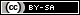This webpage is licensed under a Creative Commons Attribution-ShareAlike License.